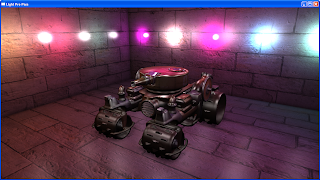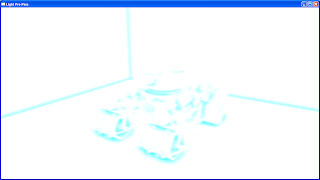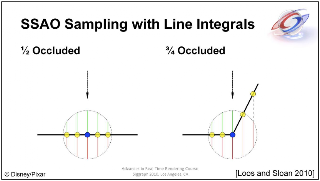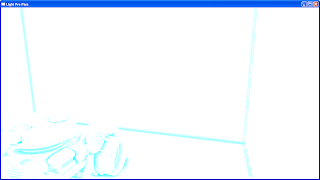### SSAO using Line Integrals

Hi everyone, this is my first post in #AltDevBlogADay. Let me introduce myself first, I am Simon Yeung, currently working as a game programmer. I like graphics programming and sometimes write iPhone apps.
This time, I would like to talk about the SSAO implemented in my little demo program. I write this demo because I spent most of my time using openGL and know little about DirectX, so I decided to learn DX by writing this demo. So it is not well optimized.
SSAO, short for Screen Space Ambient Occlusion, is a technique for approximating the indirect shadow casted by surrounding scene geometry which is done in screen space by sampling from the depth buffer.
The SSAO is implemented using the line integrals from "Rendering techniques in Toy Story 3". Here is my results:With SSAOwithout SSAOSSAO texture

Their method calculates the volume occluded by other objects inside a sphere at each fragment by sampling from the depth buffer.From Slide 22 of the paper
The volume of sphere is found by using the equation:From Slide 51 of the paper
And they use the Voronoi Diagram to associate the ratio of volume occupied by each sample points for their predefined sampling pattern.
But in my implementation, I didn't use the Voronoi Diagram, in stead, I tried to calculate the volume occupied by the depth sample using that equation in the pixel shader. However , due to the perspective projection, that equation no longer holds as the ray will not form a right angle triangle which is not the same as above figure, and resulting the artifact as below(the wall on the right side):The artifact on the wall on the right side
So, I tried to solve the problem by using ray-sphere intersection to calculate a more accurate line integrals.
For example, when calculate the occlusion volume for the black cross in the above diagram (take 2 depth samples for easy explanation), I need to compute the length L1 and L2 by solving ray-sphere intersection. Also the length O1 and O2 can be computed by sampling from the depth buffer. Therefore, the volume of the sphere can be approximated by L1+L2 and the occlusion volume can be approximated by O1+O2. (I also added a distance attenuation factor to O1 and O2 if the depth difference is too large so that the tank does not occlude the wall in my demo program). And the AO value will be (O1+O2)/(L1+L2)
This eliminate the artifacts:Solving ray-sphere intersection to eliminate the artifacts
The demo program uses 8 depth samples for each fragment. In order to fake a higher sample count, I also tried to rotate the sample points as suggested by the paper which gives a softer look for the AO:Rotating the sample points
Then, a bilateral blur is applied to smooth out the noise. Although bilateral blur is not separable, it is faster to divided it into 2 passes (i.e. 1 horizontal and 1 vertical, just like Gaussian blur), with 5 samples for each pass, which gives a softer result:After bilateral blur
Finally the SSAO texture is blend with the scene:Applying SSAO to the scene
In conclusion, I finished the SSAO but it is not optimized, there are several places can be improved such as when calculating the line integrals, I made several branches in pixel shader which slow down a lot. Also I rotated the sample points by calculate a rotation angle using the fragment position in pixel shader which can also be optimized using a pre-computed rotation angle texture as the paper suggested. These things can be improved but my main purpose of this demo is make me familiar with DirectX, so I just left out the optimization and left as future enhancement.

References:
: Rendering techniques in Toy Story 3, http://advances.realtimerendering.com/s2010/index.html